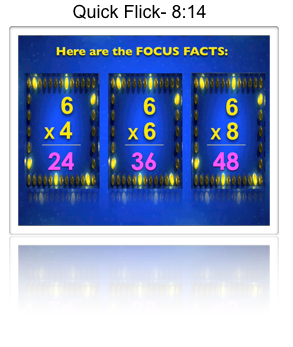##### Print or Share Flyer## 25 Aug Multiplication trick makes the Sixes facts simple!### Simplify the Sixes facts with the Half-Whole Trick!

This Multiplication trick makes learning the Sixes facts simple! The “Half-Whole” multiplication trick works like this:

### When multiplying six by an even number, the product is HALF of that number (tens place), then the WHOLE number (ones place).

Here’s an example:

## 6 X 4 = 24

### What is HALF of four? 2 (tens place) What’s the WHOLE number? 4 (ones place) So, 6X4 = 24.### Watch the instructional video (or better yet, play it for your students)!

In this Factivation!® video, students are taught that the product of 4X6 is the same as that of 6X4 due to the commutative property. The “Half-Whole” trick can still be applied regardless of the positioning of the other number (the number other than the 6).
[pinit]

## How does this Multiplication trick work?

Understanding the properties of Multiplication will deepen students’ understanding of the “why” behind this simple multiplication trick. For students who have not yet been exposed to the Distributive and Associative Property, we would suggest this very short, but entertaining video from “Why-U”, (short for “Why University”). Professor Von Schmelken introduces and illustrates the properties of Multiplication.  Video in Flash format- may not play on iPad.  Please view on a computer.

## 6×4=24

Because of the Distributive Property, we know the following to be true:

## 6×4= (5+1)4 = (5×4) + (1×4)

We can now use the Associative Property to simplify the 5×4 as shown:

## (5×2)x2 + (1×4)

which results in the following:

## 24

So, any time we multiply Six by an even number, the answer is 10n/2 + n, resulting in the simple-to-learn multiplication trick that’s true every time- the Sixes “Half-Whole” trick.

### When multiplying six by an even number, the product is HALF of that number (tens place), then the WHOLE number (ones place).

Explain this to students and open the doors to algebraic thinking, then pose these questions:

### Does the trick work when multiplying six by an ODD number? Allow student partners or groups to prove why it does/does not work.

Answer: Yes, it does work with odd numbers also. Here’s how:

## 6 x 7 = 42

### Does the trick work when multiplying six by a two-digit number? Allow student partners or groups to prove why it does/does not work.

Answer: Yes, it does work when multiplying 6 by 2-digit numbers also. Here’s how: